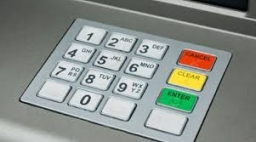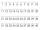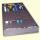# Digits

How many odd four-digit numbers can we create from digits: 0, 3, 5, 6, 7?
(a) the figures may be repeated
(b) the digits may not be repeated

n1 =  300
n2 =  54

### Step-by-step explanation:Did you find an error or inaccuracy? Feel free to write us. Thank you!

Showing 1 comment:Martin
This is OK for the number of repeat combinations.

For the number of combinations without repetition, I have the following logic:

There are 3 options (3,5,7) in the 4th position (units), so we can choose from 3 digits.
In the 1st position (thousands) there are four options (3,5,6,7), but we used one digit in the 4th position, so we can choose from 3 digits.
In the 2nd position (hundreds) there are five options (0,3,5,6,7), but in the 1st and 4th positions we have already used two digits, so we can choose from 3 digits.
In the 3rd position (tens) there are five options (0,3,5,6,7), but in the 1st, 2nd and 4th positions we have already used three digits, so we can choose from 2 digits.
Calculation: 3x3x3x2 = 54.

Breaking down all the options would give the number of numbers for numbers starting with an odd number of 12 options, and for a number starting with 6 it is 18 options, ie 12x3 + 18 = 54. If we allowed the number to start at 0, it would be another 18 possibilities, which together would be 12x3 + 18x2 = 72, but if there were 0 instead of thousands, it would be three-digit numbers.Tips to related online calculators
Would you like to compute count of combinations?

## Related math problems and questions:

• Three digits number 2Find the number of all three-digit positive integers that can be put together from digits 1,2,3,4 and which are subject to the same time has the following conditions: on one positions is one of the numbers 1,3,4, on the place of hundreds 4 or 2.
• Phone numbersHow many 7-digit telephone numbers can be compiled from the digits 0,1,2,..,8,9 that no digit is repeated?
• How manyHow many double-digit numbers greater than 30 we can create from digits 0, 1, 2, 3, 4, 5? Numbers cannot be repeated in a two-digit number.
• Dd 2-digit numbersFind all odd 2-digit natural numbers compiled from digits 1; 3; 4; 6; 8 if the digits are not repeated.
• PermutationsHow many 4-digit numbers can be composed of numbers 1,2,3,4,5,6,7 if: and the digits must not be repeated in the number b, the number should be divisible by five, and the numbers must not be repeated c, digits can be repeated
• Three digits numberFrom the numbers 1, 2, 3, 4, 5 create three-digit numbers that digits not repeat and number is divisible by 2. How many numbers are there?
• The largest numberFind the largest integer such that: 1. No figures is not repeat, 2. multiplication of every two digits is odd, 3. addition all digits is odd.
• Five-digitFind all five-digit numbers that can be created from numbers 12345 so that the numbers are not repeated and then numbers with repeated digits. Give the calculation.
• SalamiHow many ways can we choose 5 pcs of salami if we have 6 types of salami for 10 pieces and one type for 4 pieces?
• DigitsHow many five-digit numbers can be written from numbers 0.3,4, 5, 7 that is divided by 10, and if digits can be repeated?
• Three-digit numbersWe have digits 0,1,4,7 that cannot be repeated. How many three-digit numbers can we write from them? You can help by listing all the numbers.
• Divisible by fiveHow many different three-digit numbers divisible by five can we create from the digits 2, 4, 5? The digits can be repeated in the created number.
• Four-digit numbersHow many four-digit numbers can we make from the numbers 2, 6, 3, 5, 1 and 9 if the numbers in the number cannot be repeated?
• Permutations with repetitionsHow many times the input of 1.2.2.3.3.3.4 can be permutated into 4 digits, 3 digits and 2 digits without repetition? Ex: 4 digits = 1223, 2213, 3122, 2313, 4321. . etc 3 digits = 122.212.213.432. . etc 2 digits = 12, 21, 31, 23 I have tried permutation fo
• Three-digit numbersHow many are all three-digit numbers that are made up of digits 0,2,5,7 and are divisible by 9 if the digits can be repeated?
• Logik gameLetter game Logik is a two player game, which has the following rules: 1. The first player thinks five-letter word in which no letter is not repeated. 2. The second player writes a five-letter word. 3. The first player answers two numbers - the first numb
• By sixFrom the digits 1,2,3,4 we create the long integer number 123412341234. .. .. , which will have 962 digits. Is this number divisible by 6?